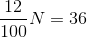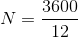Scan QR code or get instant email to install app

Question:

# 12% of a number N is 36. What is the value of N?

A 300
explanation

1212If 12% of N is 36, it means that if N is multiplied by 0.12, the answer is 36. Put this in the form of an equation and solve. Use the fraction equivalent of 12% because simplifying will make the arithmetic easier.12N = 3600 both sides multiplied by 100Both sides divided by 12

N = 300